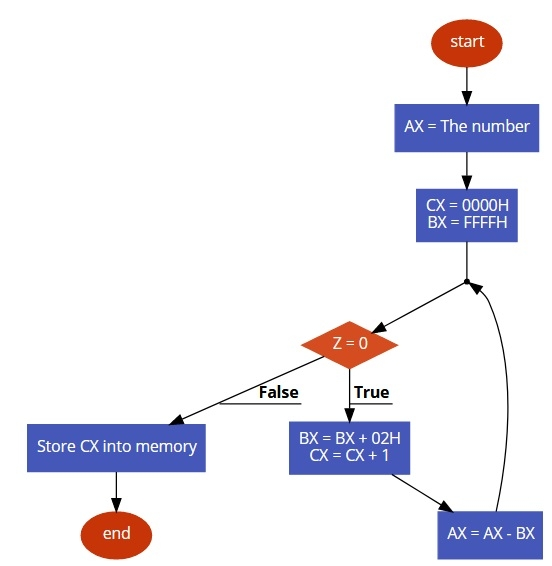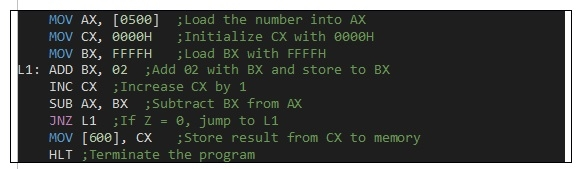# 8086 program to find Square Root of a number

In this program we will see how to find the square root of a number.

## Problem Statement

Write 8086 Assembly language program to find the square root of a number. The number is stored at memory offset 500. Finally store the result at memory offset 600.

## Discussion

To find the square root here at first we are clearing the counter register. In each iteration we are increasing BX register by 2. At first we need BX = 0001. So we are initializing it to FFFFH, after adding 2, it will be 0001H. In each iteration the counter value is increased, and subtract the BX value from AX, until the AX is 0, the loop will run.

Data

500
90

## Flow Diagram## Program## Output

Data

600
90

Updated on: 30-Jul-2019

2K+ Views